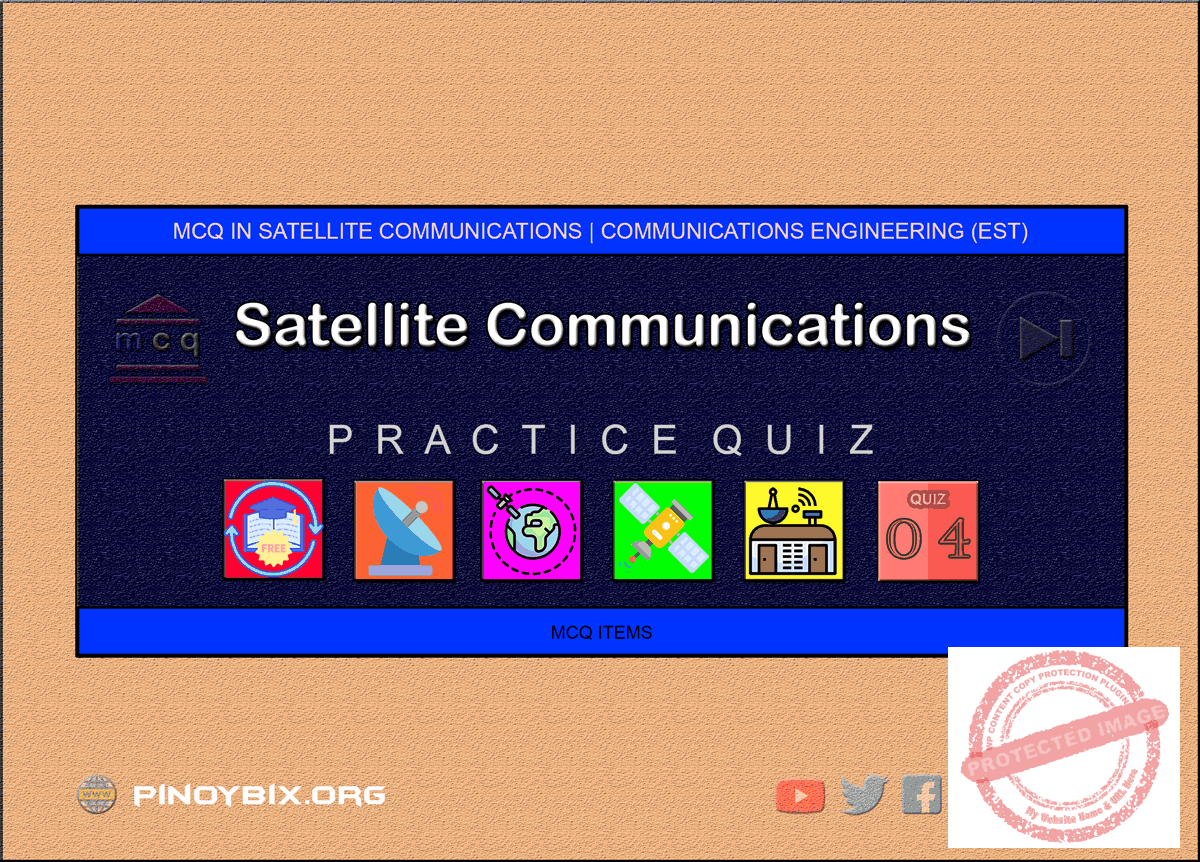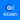# MCQ in Satellite Communications Part 4 | ECE Board Exam

(Last Updated On: May 3, 2020)This is the Multiples Choice Questions Part 4 of the Series in Satellite Communications as one of the Communications Engineering topic. In Preparation for the ECE Board Exam make sure to expose yourself and familiarize in each and every questions compiled here taken from various sources including but not limited to past Board Examination Questions in Electronic System and Technologies, Communications Books, Journals and other Communications References.

#### MCQ Topic Outline included in ECE Board Exam Syllabi

• MCQ in Satellite System
• MCQ in Types of Satellite
• MCQ in Satellite Orbit
• MCQ in Demand Assignments Multiple Access
• MCQ in Antenna Tracking
• MCQ in Satellite Link Budgets
• MCQ in Satellite Path Loss
• MCQ in Satellite Figure of Merit
• MCQ in Ratio of Carrier to thermal Noise Power
• MCQ in Station Margin
• MCQ in VSAT

#### Continue Practice Exam Test Questions Part 4 of the Series

151. A common up-converter and down-converter IF in satellite communications is

A. 36 MHz

B. 40 MHz

C. 70 MHz

D. 500 MHz

Solution:

152. It is the angle between the earth’s equatorial plane and the orbital plane of the satellite measured counterclockwise.

A. Angle of elevation

B. Angle of azimuth

C. Angle of inclination

D. Angle of tetrahedron

Solution:

153. Which of the following types of HPA is not used in earth stations?

A. TWT

B. Klystron

C. Transistors

D. Magnetron

Solution:

154. Galileo satellite’s mass is ____.

A. 880 kg

B. 675 kg

C. 900 kg

D. 540 kg

Solution:

155. The point where a polar or inclined orbit crosses the equatorial plane traveling from north to south

A. Ascending node

B. Line of nodes

C. Descending node

D. Diagonal nodes

Solution:

156. The receive GCE system in an earth station performs what function/s

A. Modulation and multiplexing

B. Up conversion

C. Demodulation and demultiplexing

D. Down conversion

Solution:

157. The term generally associated with the table showing the position of a heavenly body on the number of dates in a regular sequence.

A. Astronomical almanac

B. Smith

C. Ephemeris

D. Space reporting

Solution:

158. The point where a polar or inclined orbit crosses the equatorial plane traveling from south to north.

A. Ascending node

B. Descending node

C. Diagonal node

D. Inclined node

Solution:

159. The line joining the ascending and descending node is called ____.

A. Line of apsides

B. Line of nodes

C. Line of shoot

D. Any of these

Solution:

160. When the satellite rotates in an orbit directly above the equator, usually in circular pattern. This type of orbit is called ____.

A. Polar orbit

B. Synchronous orbit

C. Geosynchronous satellite

D. Equatorial orbit

Solution:

161. The Navstar GPS satellite system was declared fully operational as of ____.

A. April 17, 1995

B. April 24, 1995

C. April 27, 1995

D. April 10, 1995

Solution:

162. The HPAs in most satellites are

A. TWTs

B. Vacuum tubes

C. Klystrons

D. Magnetrons

Solution:

163. The physical location of a satellite is determined by its

A. Distance from the earth

B. Latitude and longitude

C. Reference to the stars

D. Position relative to the sun

Solution:

164. When the satellite rotates in a path that takes it over the north and south poles in an orbit perpendicular to the equatorial plane. This type of orbit is called ____.

A. Inclined orbit

B. Polar orbit

C. Geosynchronous orbit

D. Diagonal orbit

Solution:

165. The azimuth angles and the angle of elevation is collectively known as ____.

A. Antennas look angles

B. Antennas see angles

C. Antennas keep angles

D. Antennas satellite angles

Solution:

166. The point on the surface of the Earth directly below the satellite.

A. Satellite point

B. Latitude

C. Longitude

D. Subsatellite point

Solution:

167. It is the vertical angle formed between the direction of travel of an electromagnetic wave radiated from an earth station antenna pointing directly toward a satellite and the horizontal plane.

A. Angle of depression

B. Angle of inclination

C. Angle of elevation

D. Angle of azimuth

Solution:

168. The unique number used to encrypt the signal from that of the GPS satellite.

A. SV

B. UV

C. PRN

D. ESN

Solution:

169. The minimum acceptable angle of elevation

A. 6 degrees

B. 4 degrees

C. 5 degrees

D. 7 degrees

Solution:

170. It is the horizontal angular distance from a reference direction either the southern or northern most point of the horizon.

A. Angle of elevation

B. Latitude

C. Longitude

D. Azimuth

Solution:

171. It is defined as the horizontal pointing angle of an earth station antenna.

A. Angle of inclination

B. Azimuth angle

C. Latitude

D. Longitude

Solution:

172. Determines the farthest satellite away that can be seen looking east or west of the earth station’s longitude

B. Optical horizon

C. Terrestrial limits

D. Limits of visibility

Solution:

173. The spatial separation of a satellite is between ____.

A. 3 to 6 degrees

B. 1 to 2 degrees

C. 5 to 8 degrees

D. 8 to 16 degrees

Solution:

174. The geographical representation of a satellite antenna’s radiation pattern is called a ____.

A. Field intensity

B. Footprint

D. Polarization

Solution:

175. The GPS satellite identification which is assigned according to the order of the vehicle’s launch.

A. SV

B. EV

C. PRN

D. ESN

Solution:

176. The smallest beam of a satellite that concentrates their power to very small geographical areas.

A. Hemispherical beam

B. Global beam

C. Spot beam

D. Any of these

Solution:

177. Satellite’s radiation pattern that typically target up to 20% of the Earth’s surface.

A. Hemispherical beam

B. Global beam

C. Spot beam

D. Any of these

Solution:

178. The key electronic component in a communications satellite is the

A. Telemetry

B. Command and Control system

C. On-board computer

D. Transponder

Solution:

179. The radiation pattern that has a satellite’s antenna beamwidth of 17 degrees and are capable of covering approximately 42% of the earth’s surface.

A. Hemispherical beam

B. Earth beam

C. Spot beam

D. Any of these

Solution:

180. The first active satellite

A. Moon

B. Sputnik I

C. Score

D. Echo

Solution:

181. Sputnik I transmitted telemetry information for

A. 1 week

B. 21 days

C. 15 days

D. 30 days

Solution:

182. The US counterpart of Sputnik I

A. Syncom

B. Telstar

C. Echo

D. Explorer I

Solution:

183. Explorer I lasted for ____.

A. 5 months

B. 6 months

C. 12 months

D. 2 months

Solution:

184. Navstar GPS satellite grouping that can operate continuously for 180 days between uploads from the ground.

A. Block III

B. Block IIa

C. Block II

D. Block IIR

Solution:

185. It was the first artificial satellite used for relaying terrestrial communications

A. Score

B. Explorer I

C. Sputnik I

D. Syncom I

Solution:

186. Which of the following satellite rebroadcast President Eisenhower’s 1958 Christmas message?

A. Telstar I

B. Telstar II

C. Echo

D. Score

Solution:

187. The satellite or space segment of Navstar GPS is consist of ____ operational satellites.

A. 30

B. 24

C. 14

D. 20

Solution:

188. It was the first satellite to accomplish transatlantic transmission.

A. Courier

B. Echo

C. Telstar

D. Syncom

Solution:

189. It is the first transponder-type satellite

A. Telstar I

B. Sputnik I

C. Syncom

D. Courier

Solution:

190. The first active satellite to simultaneously receive and transmit radio signals.

A. Telstar I

B. Telstar II

C. Intelsat

D. Syncom

Solution:

191. The satellite communications channel in a transponder are defined by the

A. LNA

B. Bandpass filter

C. Mixer

D. Input signals

Solution:

192. The satellite that was damaged by radiation from a newly discovered Van Allen Belts and consequently lasted for two weeks.

A. Telstar I

B. Telstar II

C. Intelsat

D. Syncom

Solution:

193. As the height of a satellite orbit gets lower, the speed of the satellite

A. Increases

B. Decreases

C. Remains the same

D. None of the above

Solution:

194. The satellite that was lost in space in its first attempt to place it in a geosynchronous orbit.

A. Syncom I

B. Telstar I

C. Telstar II

D. Courier

Solution:

195. Intelsat I which was the first commercial telecommunications satellite is called ____.

A. Domsat

B. Molniya

C. Early bird

D. Courier

Solution:

196. Block II Navstar GPS satellites can operate for approximately ____ between the receiving updates and corrections from the control segment of the system.

A. 5.5 days

B. 3.5 days

C. 6 days

D. 7 days

Solution:

197. The angle of inclination of a satellite in polar orbit is nearly ____.

A. 45 degrees

B. 90 degrees

C. 30 degrees

D. 60 degrees

Solution:

198. It is the time it takes earth to rotate back to the same constellation

A. Revolution

B. Rotation

C. Sidereal period

D. Year

Solution:

199. Satellites with orbital pattern like Molniya are sometimes classified as

A. LEO

B. MEO

C. GEO

D. HEO

Solution:

200. The process of maneuvering a satellite within a preassigned window is called

A. Satellite keeping

B. Station controlling

C. Station keeping

D. Satellite controlling

Solution:

#### Questions and Answers in Satellite Communications Series

Following is the list of multiple choice questions in this brand new series:

MCQ in Satellite Communications
PART 1: MCQ from Number 1 – 50                        Answer key: PART 1
PART 2: MCQ from Number 51 – 100                   Answer key: PART 2
PART 3: MCQ from Number 101 – 150                 Answer key: PART 3
PART 4: MCQ from Number 151 – 200                 Answer key: PART 4
PART 5: MCQ from Number 201 – 250                 Answer key: PART 5
PART 6: MCQ from Number 251 – 300                 Answer key: PART 6
PART 7: MCQ from Number 301 – 350                 Answer key: PART 7

### Complete List of MCQ in Communications Engineering per topic

Help Me Makes a Difference!

 P inoyBIX educates thousands of reviewers/students a day in preparation for their board examinations. Also provides professionals with materials for their lectures and practice exams. Help me go forward with the same spirit. “Will you make a small gift today via GCASH?”+63 966 459 6474 Option 1 : \$1 USD Option 2 : \$3 USD Option 3 : \$5 USD Option 4 : \$10 USD Option 5 : \$25 USD Option 6 : \$50 USD Option 7 : \$100 USD Option 8 : Other Amount© 2014 PinoyBIX Engineering. © 2019 All Rights Reserved | How to Donate? |